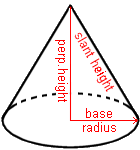Cleave Books
The Cones Calculator
 Show values to . . . 3 4 5 6 7 8 9 significant figures.
 base radius = units perp. height = unitsslant height = units C S A = square units volume = cubic units *S* angle = degrees
Remember: Appropriate units need to be attached.
C S A is curved surface area
For *S* angle see notes below.
Very large and very small numbers appear in e-Format.
Unvalued zeros on all numbers have been suppressed.
The original inputs have NOT been adjusted in any way.
A note on Format and Accuracy is available.

 Additional Information A cone is a often seen in the real world of ice-cream and traffic cones; as well as the fantasy world of witches and wizards where it commonly used for their hats.Its shape is formed by having a circle at one end (usually referred as the base) and then tapering to a point at the other end. This point is the apex.The line from the centre of the base circle to the apex is the perpendicular height. This is different from the slant height which is any one of the straight lines running from a point on the edge of the base circle to the apex. Notice, in the diagram above, how the perp. height, slant height and base radius form a right-angled triangle. This is often useful when solving problems about the cone.More correctly this should be described as a 'right circular-based cone' because the base is a circle (it could be some other shape) and because the apex is on the right-perpendicular above the centre of that circle. But usually it is just called a cone. The *S* angle.The easiest way to make a cone is by cutting a sector out of a circle and then folding it around so that the two cut edges meet. To make a cone of a particular size it is necessary to know the radius of the circle from which the sector is to be cut, and also the angle of that sector.Both of these pieces of information are given by the calculator. The radius is the same as the slant height, and the angle is shown as the *S* angle.The sector used to make the cone is also known as the development of that cone. The area of that sector is the curved surface area of the cone.CautionsThe message that 'No solution was found' means only that. So, if a solution is possible then it was outside the limits set within this calculator.Also, in some cases, more than one solution might be possible, but only one (the first one found) is given here.

Go to Cleave Books main Index